# 1.头文件与类的声明

## c++关于数据的函数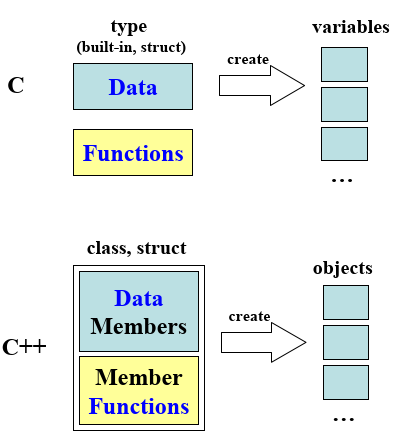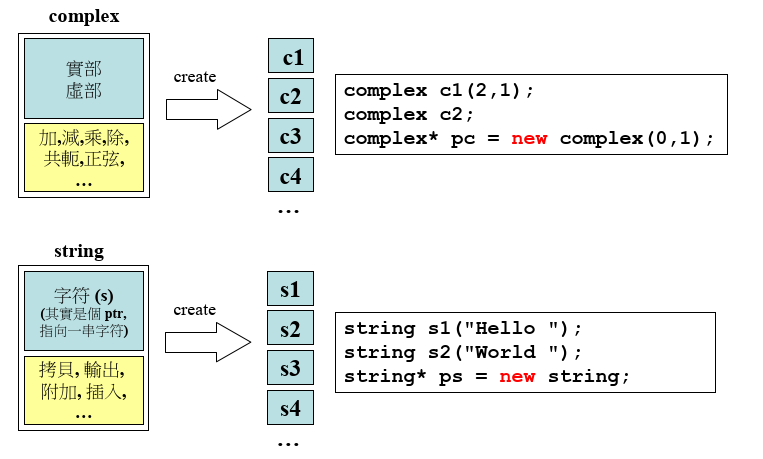## 正式开始之前：代码基本形式

c++代码主要由以下三部分组成：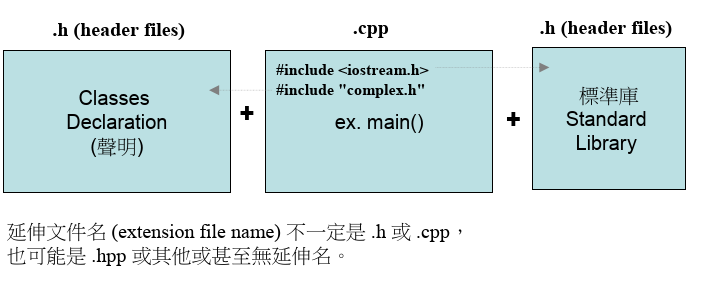## c和c++的输出的区别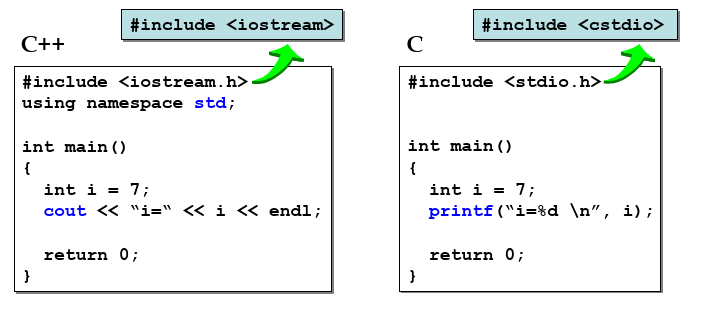c++的优势是cout啥都可以丢！

## 头文件正规写法：防御声明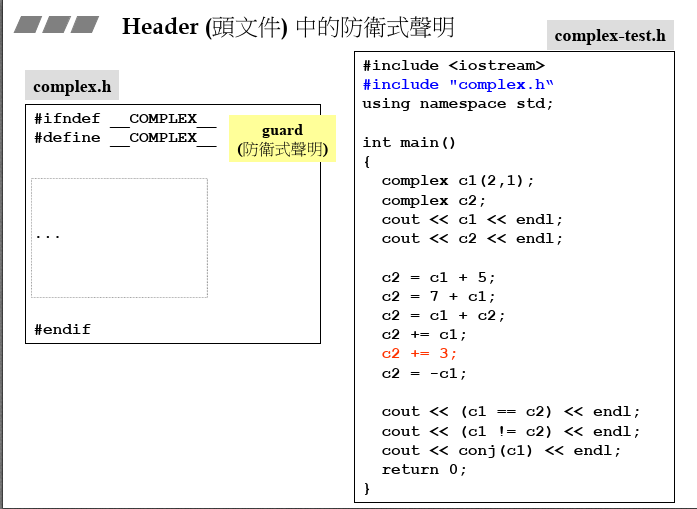## 头文件正规写法：布局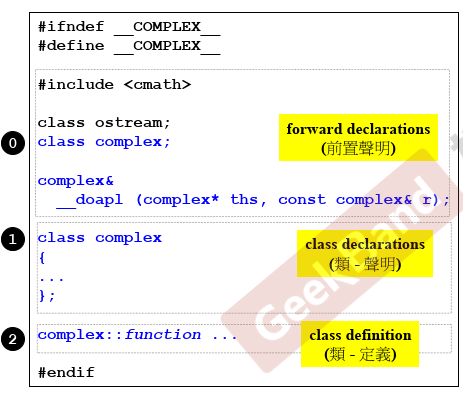## class的声明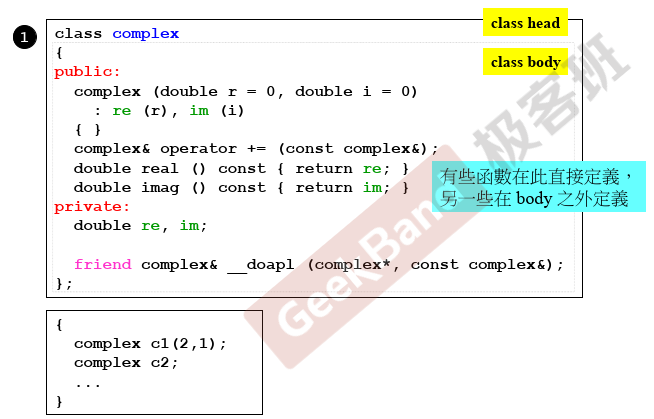## class的模板：解决重复性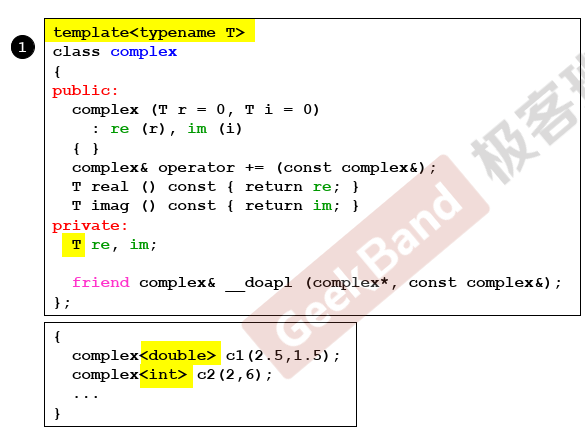T可以随意替换，第一行告诉编译器啥是T，下面的为实例指定double或者int。

# 2.构造函数

## inline函数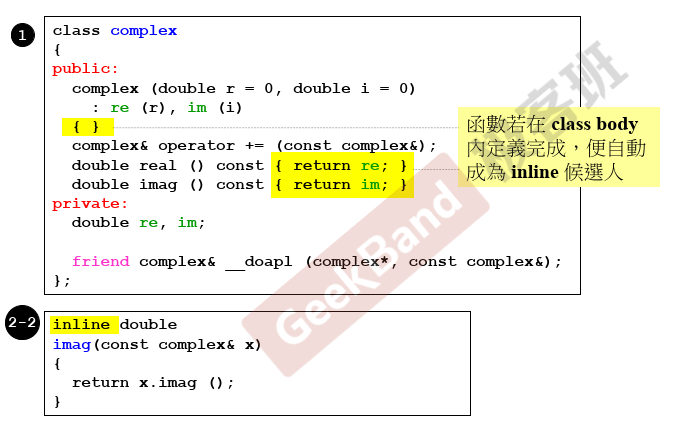inline拥有很大的优势，速度很快！但是是否inline由编译器决定！

## 访问级别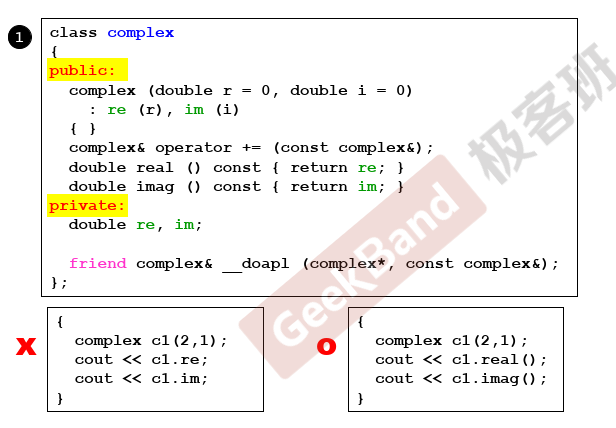public可以被外界看到，private就要封装起来！

## 构造函数是什么？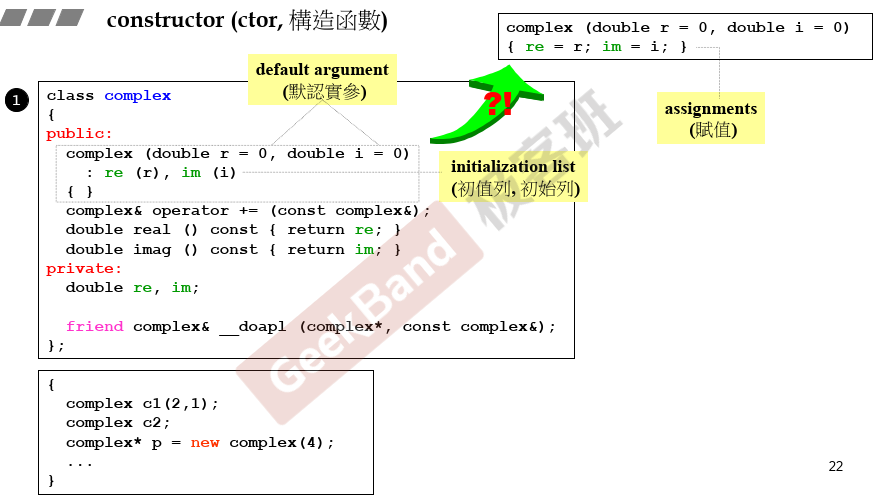``````complex (double r = 0, double i = 0)
: re (r), im (i) ``````

# 3.参数传递与返回值

## 构造函数可以有很多个-重载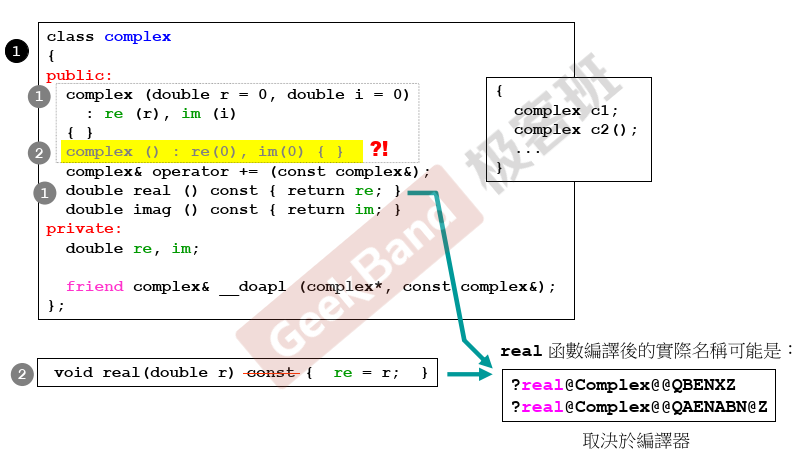## 把构造函数放在private区

``````class complex
{
public:

complex& operator += (const complex&);
double real () const { return re; }
double imag () const { return im; }
private:
double re, im;
complex (double r = 0, double i = 0)
: re (r), im (i)
{ }
friend complex& __doapl (complex*, const complex&);

};``````

``````{

complex c1(2,1);
complex c2;
...

}``````

``````class A {
public:
static A& getInstance();
setup() { ... }
private:
A();
A(const A& rhs);
...
};

A& A::getInstance()
{
static A a;
return a;
}``````

## 常量成员函数

``````class complex
{
public:
complex (double r = 0, double i = 0)
: re (r), im (i)
{ }
complex& operator += (const complex&);
double real () const { return re; } //在这里
double imag () const { return im; }
private:
double re, im;

friend complex& __doapl (complex*, const complex&);

};``````

real和imag不改变数据，则马上加上const。

``````{

const complex c1(2,1);
cout << c1.real();
cout << c1.imag();

}``````

## 返回值传递：by value or by reference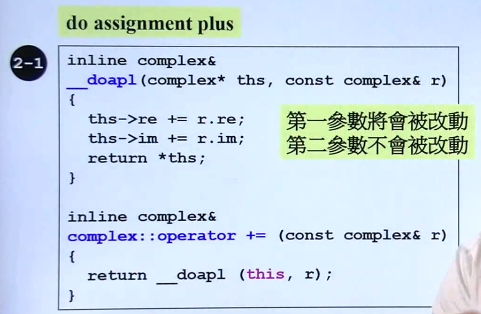## 友元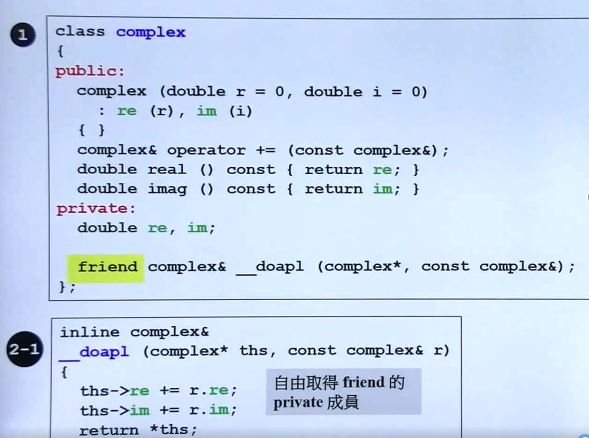# 4.操作符重载与临时对象

## 操作符重载-1，成员函数this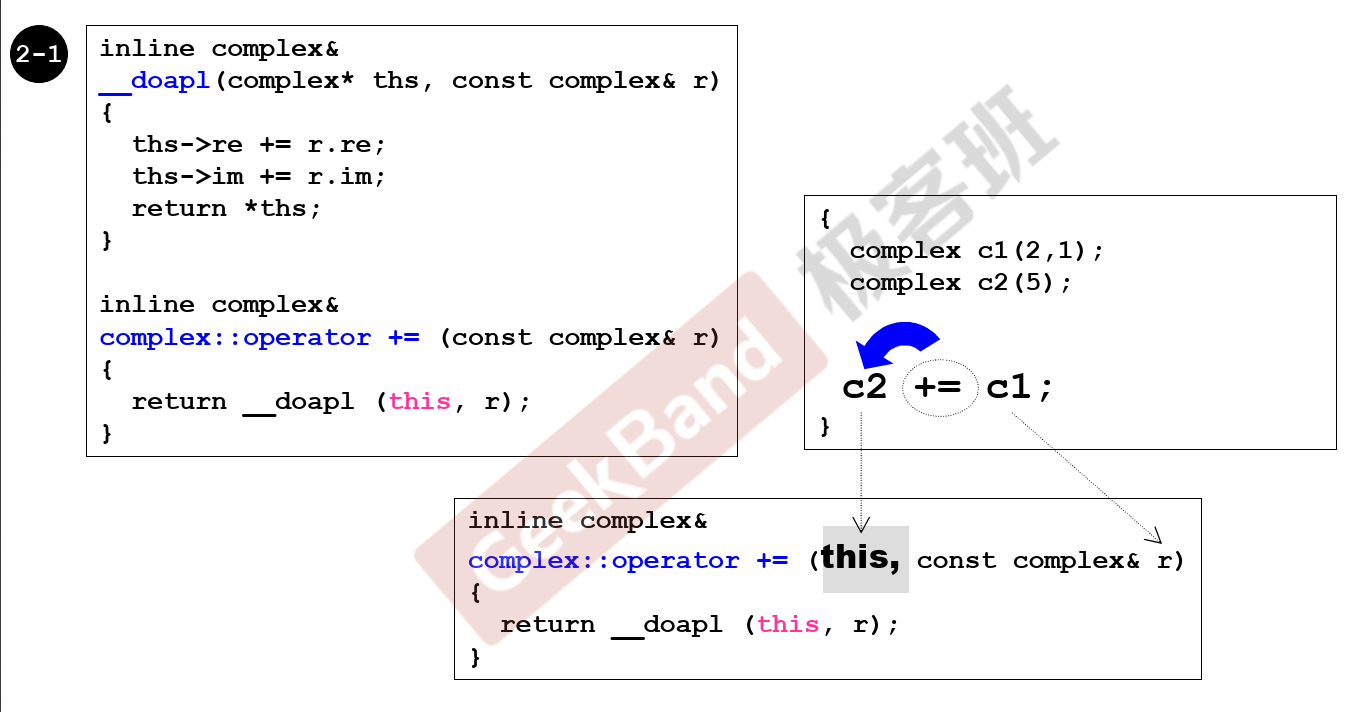c2+=c1的理解，+=作用于c2

## return by reference 语法分析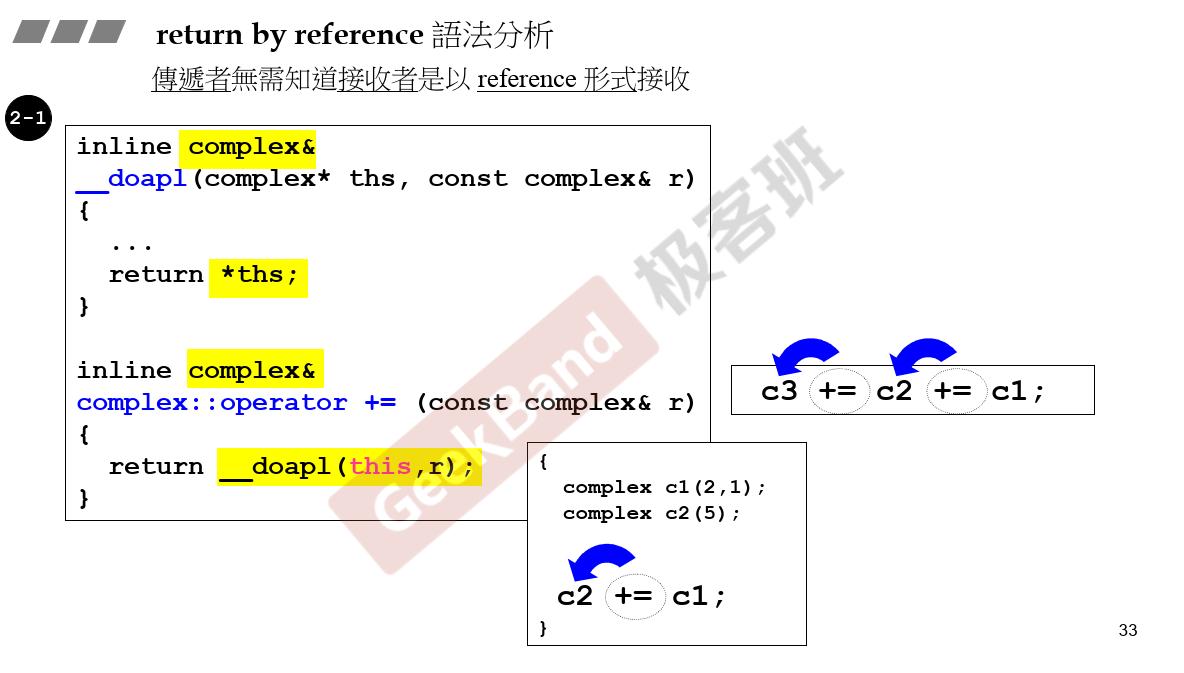## class body之外的各种定义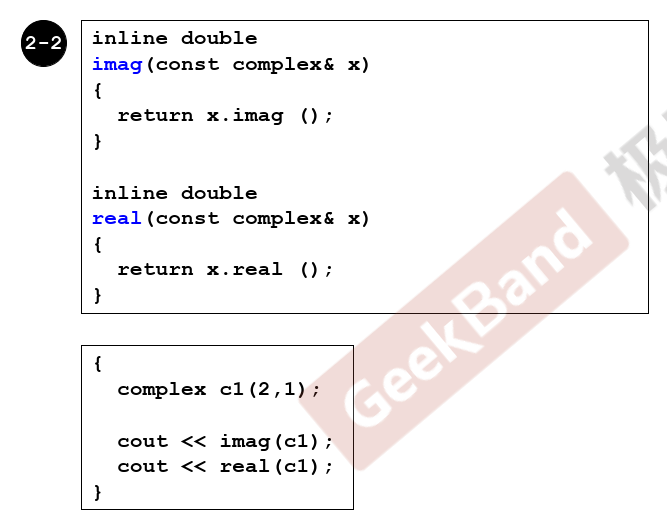## 操作符重载-2，非成员函数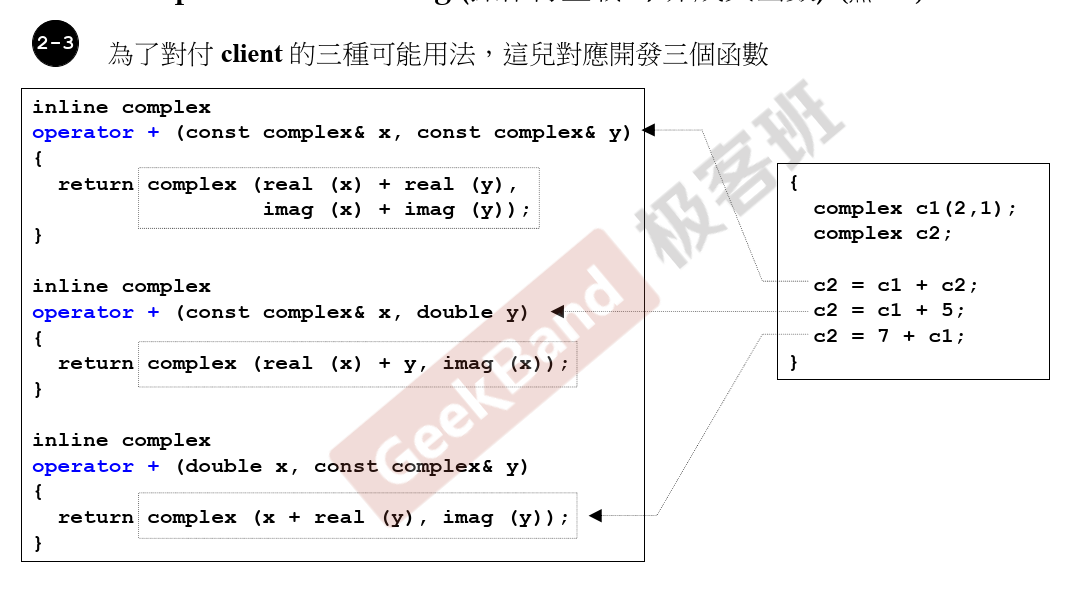## temp object(临时对象) typename()

typename生命很短，无需命名。

## 各种操作符重载，非成员函数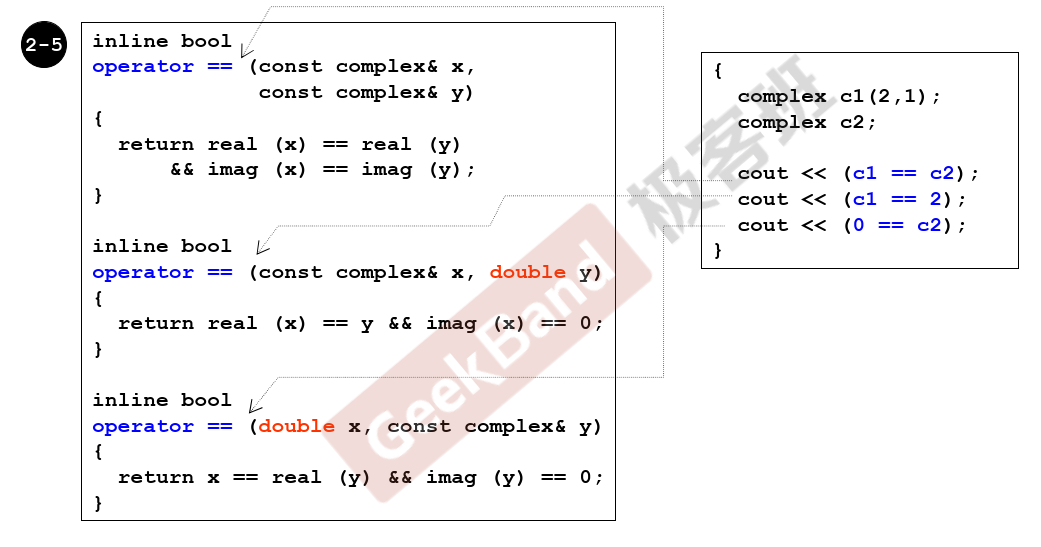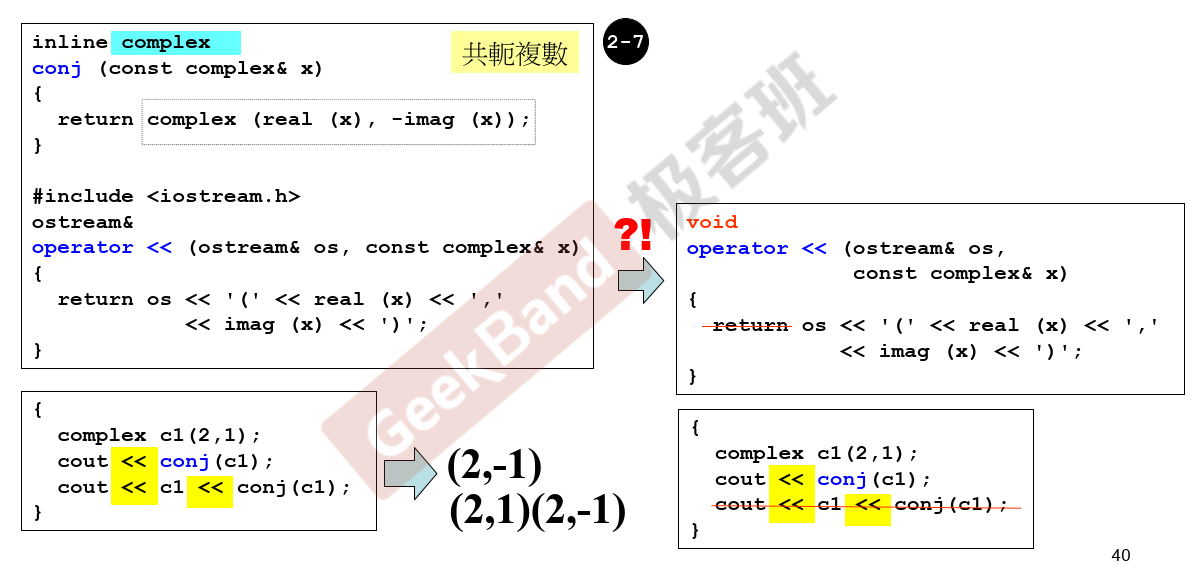# 复习

``````#ifndef _COMPLEX_
#define _COMPLEX_
class complex;
class complex& __doapl(complex*, const complex&);
class complex
{
public:
complex(double r = 0, double i = 0)
:re(r), im(i) //只需要经过一步编译就可返回，很好用！
{};
complex& operator += (const complex&);
double real() const { return re; }
double imag() const { return im; }

private:
double re, im;
friend complex& __doapl(complex*, const complex&);

};
inline complex&
__doapl(complex* ths, const complex& r)
{
ths->re += r.re;
ths->im += r.im;
return *ths;
}
//because right could be change and can abundant, so add const
inline complex& complex::operator += (const complex& r) {
return __doapl(this, r);
} //only write right

inline double
imag(const complex& x)
{
return x.imag();
}

inline double
real(const complex& x)
{
return x.real();
}

inline complex
operator + (const complex& x, const complex& y)
{
return complex (real (x) + real (y), imag (x) + imag (y));
}
//why not design  member function,because complex + true number
inline complex
operator + (const complex& x, double  y)
{
return complex(real(x) + y, imag(x));
}//why not design  member function,because complex + true number
inline complex
operator + (double x, const complex& y)
{
return complex(x + real(y), imag(y));
}//why not design  member function,because complex + true number

#include
operator << (std::ostream& os, const complex& x) //cout will change
{
return os << '(' << real(x)  << ',' << imag(x) << ')';
}
#endif``````

• 设计一个class构造函数：re(r)要用起来
• 函数加不加const一定要考虑
• 参数传递一定要考虑&
• return要不要&
• 数据尽可能的放在private，函数放在public